4. CHEMICAL KINETICS

Q. 1. Define ‘energy of activation of a reaction. How does it vary with a rise in temperature ?

Ans Energy of activation : The minimum energy over average energy which must be gained by the molecules before they could react to form products is called the energy of activation. It is denoted by Ea.

According to the Arrhenius theory, activation energy is independent of temperature. However, precise measurements indicate that the activation energy tends to decrease slightly with a rise in temperature.

Q. 2. State and explain Arrehenius equation. How can we determine the activation energy of a reaction using this equation ?

Ans⇒ k = AeEa/RT

where k is rate constant, A is Arrhenius of frequency factor, Ea is energy of activation, T is absolute temperature. This equation is called Arrhenius equation.
Taking log, we get

In k = In A –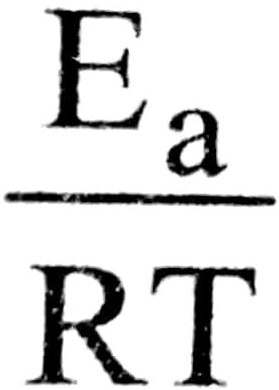2.303 log k = 2.303 log A A –log k = log A –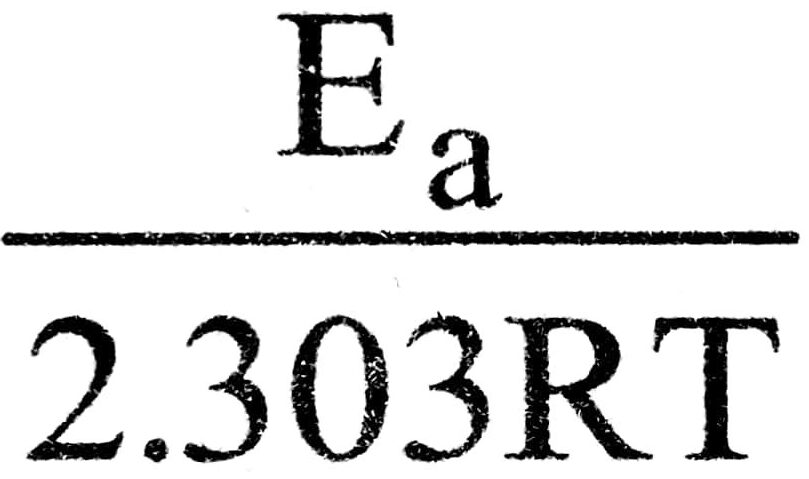Plot of log k versus 1/T is a straight line with a negative slope. The slope of the line is equal to

.                       Slope = –∴                       Ea = slope × 2.303 × R

Q. 3. Explain the relationship between the rate of production of iodine and rate of disappearance of hydrogen iodide in the following reaction ?

2HI → H2 + I2

Ans⇒ Rate of disappearance of HI =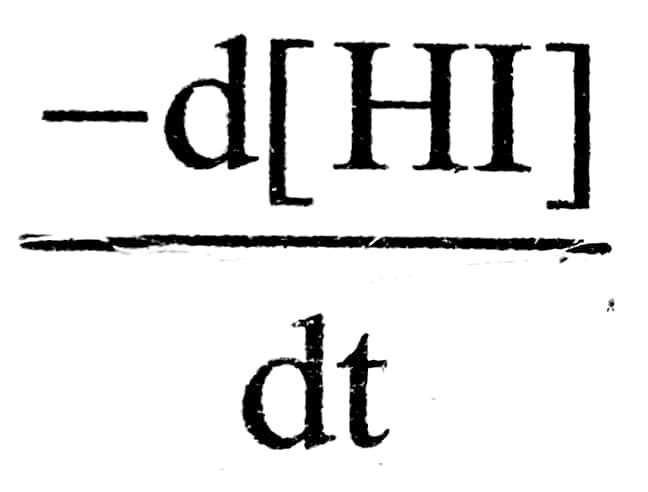Rate of appearance of I2 =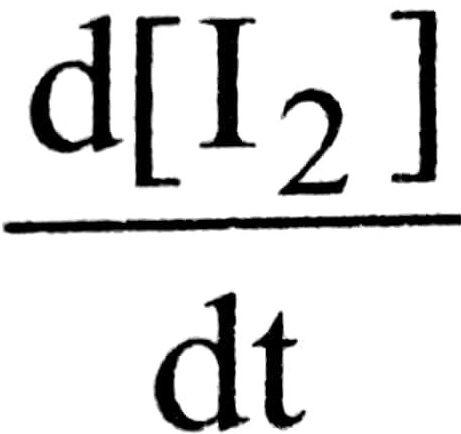From the chemical equation,

Rate of production I2 =× Rate of disappearance of HI

Therefore,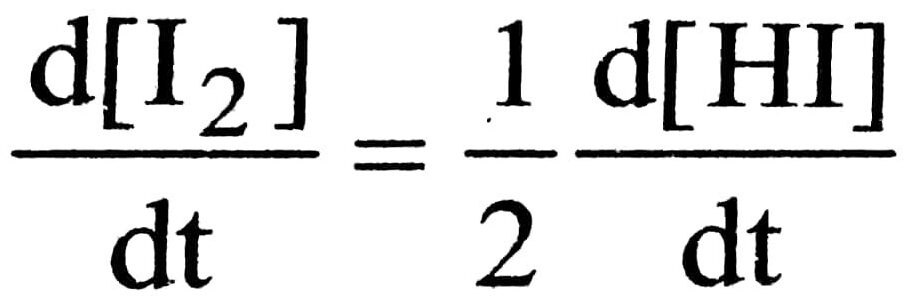Q. 4. Define Half-life period (t12) of a chemical reaction. Also obtain the expression for half-life period.

Ans⇒ Half-life period (t1/2) of a chemical reaction.

Half-life period of a chemical reaction is defined as a time period during which the concentration of a reactant is reduced to half of the initial value of concentration.
It is denoted by t1/2 or to.5.

The rate equation for a reaction of first order in expressed as :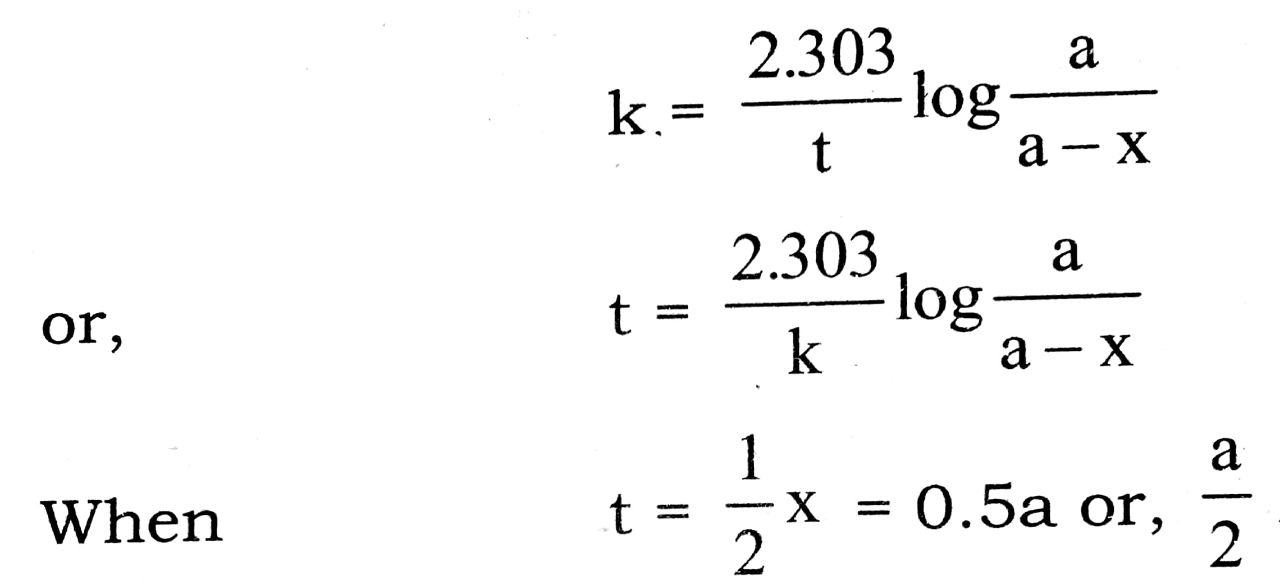On substituting these values, we get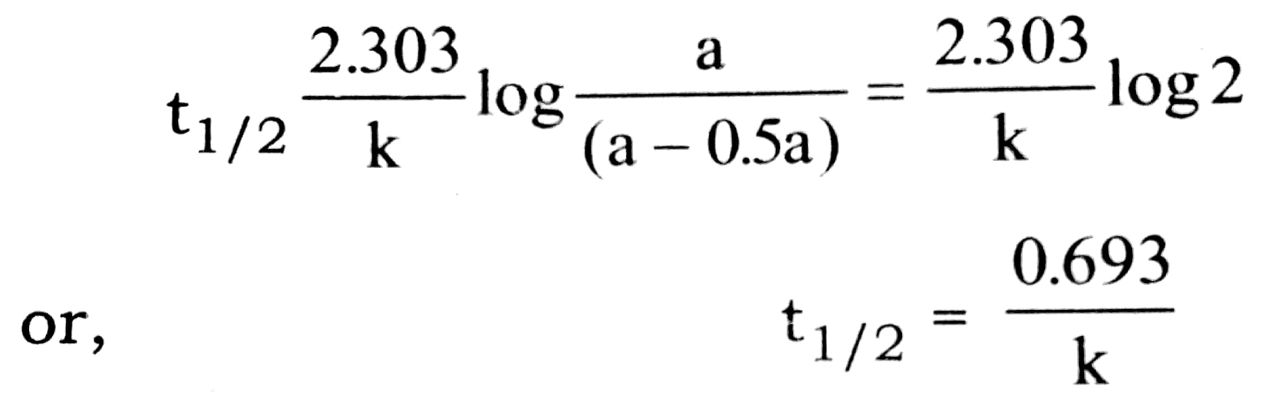The relation (i) is the required expression for half life period of first order reaction.

Q. 5. Express the rate of each of the following reaction in terms of disappearance of the reactants and appearance of products :

(i) PCl5 (g) → PCl3 (g) Cl2(g)
(ii) 2NO (g) + O2(g) → 2NO2 (g)
(iii) H2 (g) + I2 (g) → 2HI (g)
(iv) CO (g) + NO2 (g) → CO2 (g) + NO (g)
(v) 2NO2 (g) + F2 (g) → 2NO2 F(g)

Ans⇒ (i) PCl5 (g) → PCl3 (g) + Cl2 (g)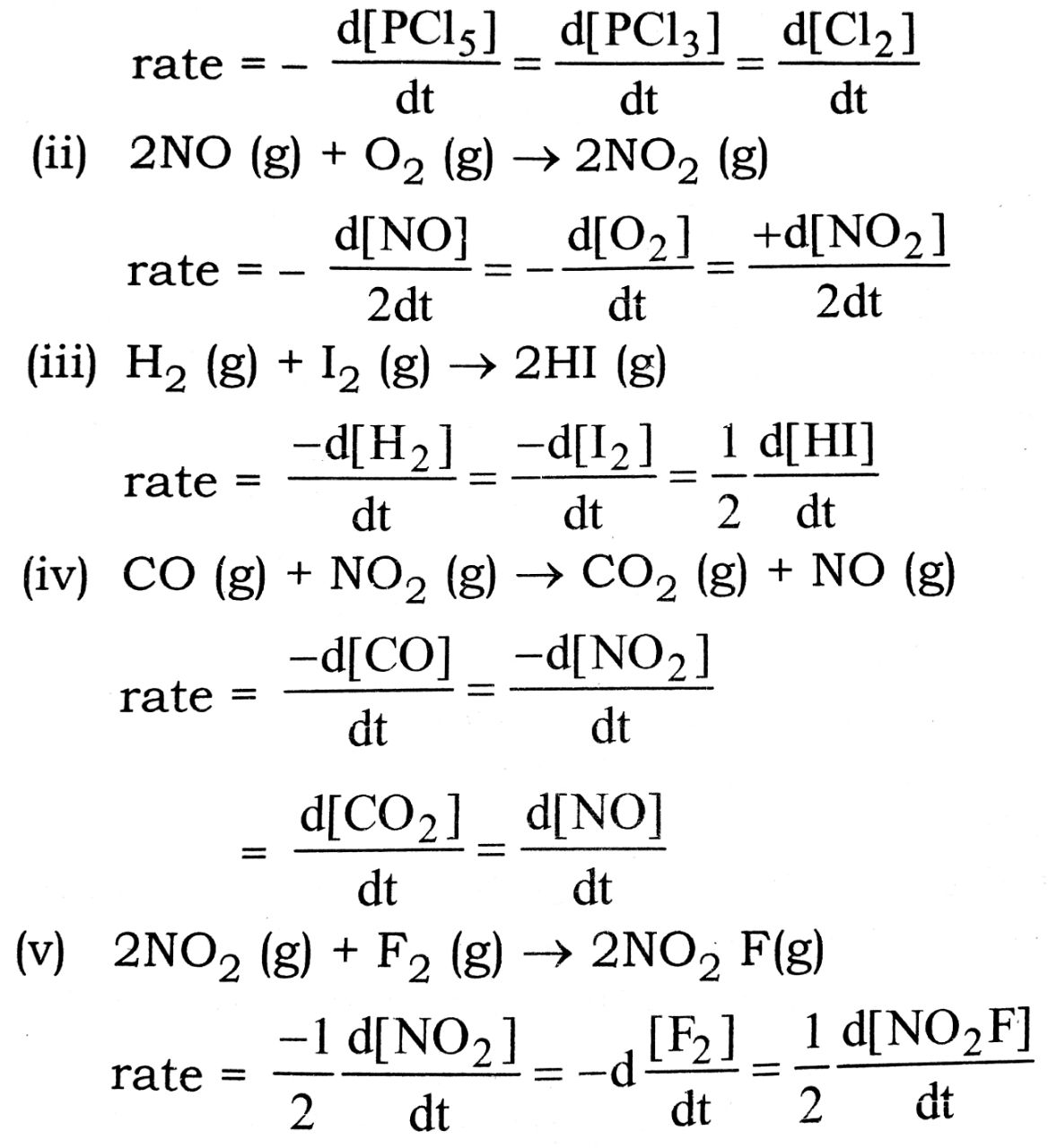Q. 6. The reaction :

2NO + Br2 → 2NOBr

is supposed to the following mechanism :

(i) NO + Br2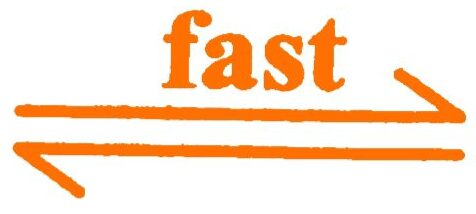NOBr2
(ii) NOBr + NO2NOBr
Suggest the rate law expression.

Ans⇒ The rate expression is derived by step II of the mechanism as it is the slower one

Rate = k[NOBr2] [NO]

(i) However, NOBr2 is an intermediate and thus its concentration should be replaced from equation (i) From step (i),

Equilibrium constant, Kc =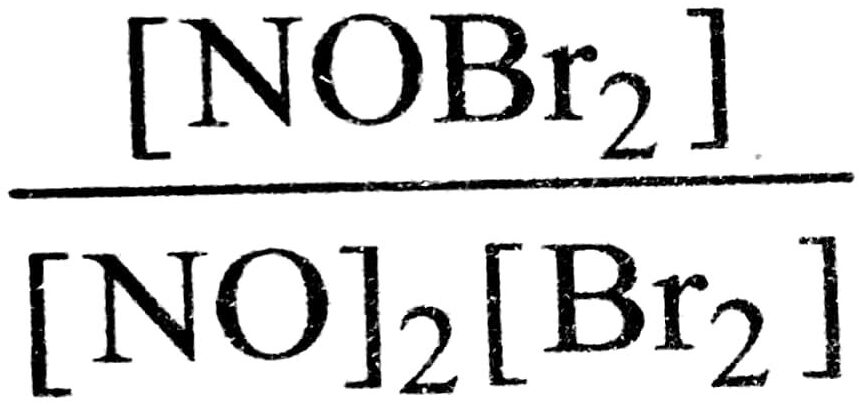∴ [NOBr2] = Kc [NO] [Br2]

Then by equation (i) and (ii)

rate = k – [NO]2 [Br2]

Q. 7. (a) Define half life period of a chemical reaction. Write equation of t1/2 for a first order reaction.

(b) A first order reaction is 75% complete in 60 minutes. Find the half life of this reaction.

Ans⇒ (a) The time during which half of the reactant is converted into the products is called half life period of the reaction. It is represented by t1/2.

tfor a first order reaction is t=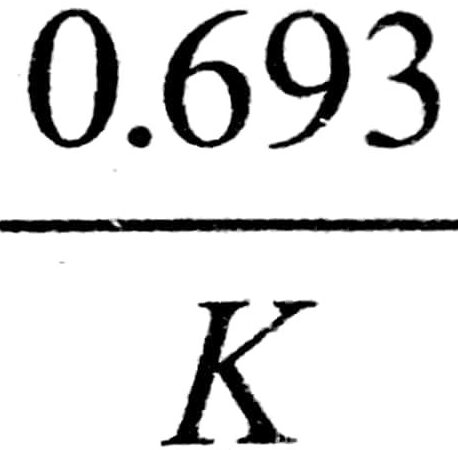(b) Let Initial concentration = [A]0

Final concentration = [A]t = [A]0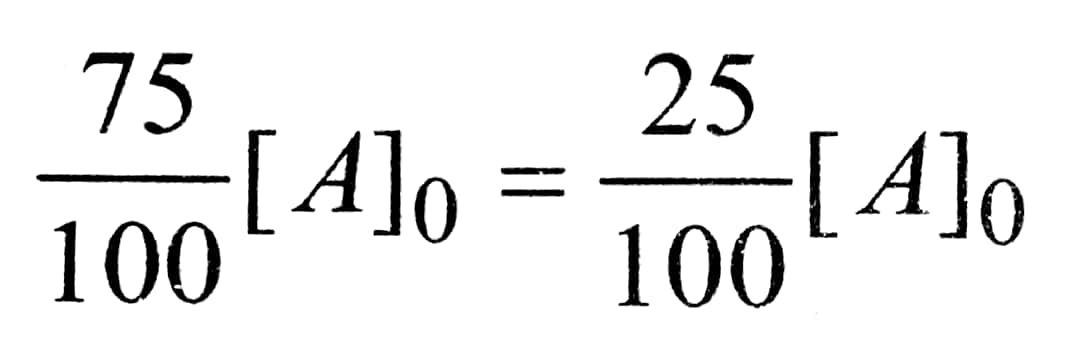Time required (t) = 60 min
Them for a first order reaction.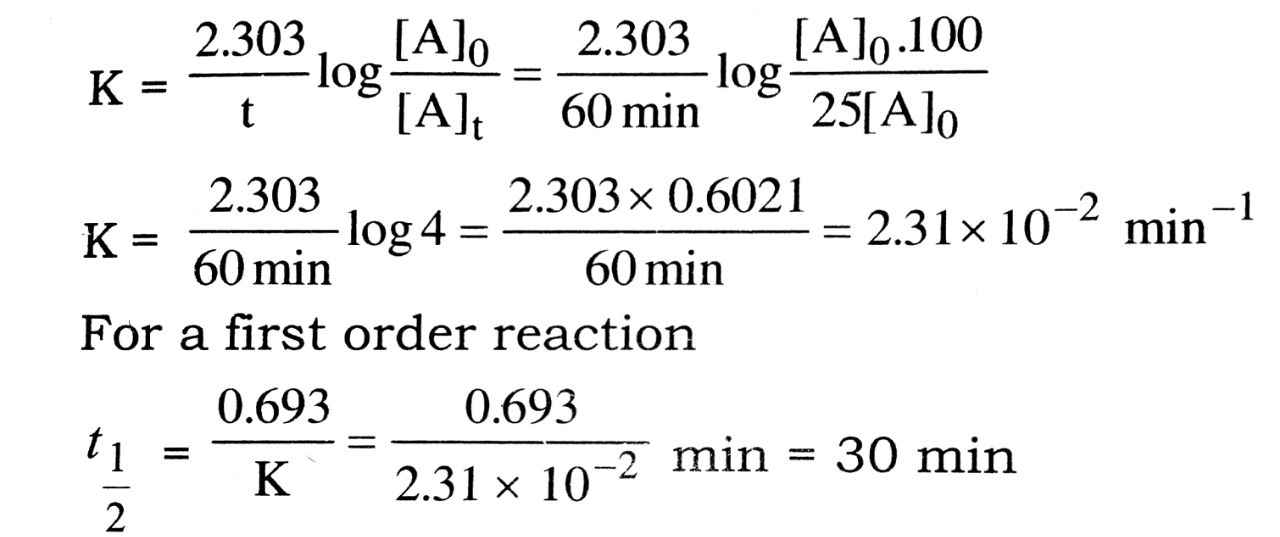Q. 8. What is the effect of temperature on the rate constant of reaction ? How can this temperature effect on rate constant be represented quantitatively.

Ans⇒ The rate constant of reaction increases with increase of temperature. This increase in generally fold to five two fold for 10 K rise in temperature. This is explained on the basis of collision theory. The main parts of collision theory are as follows :

(i) For reaction to occur, there must be collision between the reacting species.
(ii) Only a certain fraction of total collisions are effective in forming the products.
(iii) For effective collisions, the molecule must possess the sufficient energy (equal or greater than threshold energy) as well as proper orientation.

On the basis above conclusions, the rate of reaction is given by
Rate = f × 2 (where fis the effective collisions and is total number of collisions per unit volume per second)
Quantitatively, the effect of temperature on the rate of a reaction and hence on the rate constant k was proposed by Arrhenius The equation called Arrehenius equation is usually written in the form

K = Ae-Ea/RT

where A is a constant called frequency factor (because it gives the frequency of binary collisions of the reacting molecules per second per litre. E0 is the energy of activation, R is a gas constant and T is the absolute temperature. The factor e-Ea/RT gives the fraction of molecules having energy equal to or greater than the activation energy, Ea.

The energy of activation (Ea) is an important quantity and it is characteristic of the reaction. using the above equation, its value can be calculated.

Taking logarithm or both sides of equation (i), we get,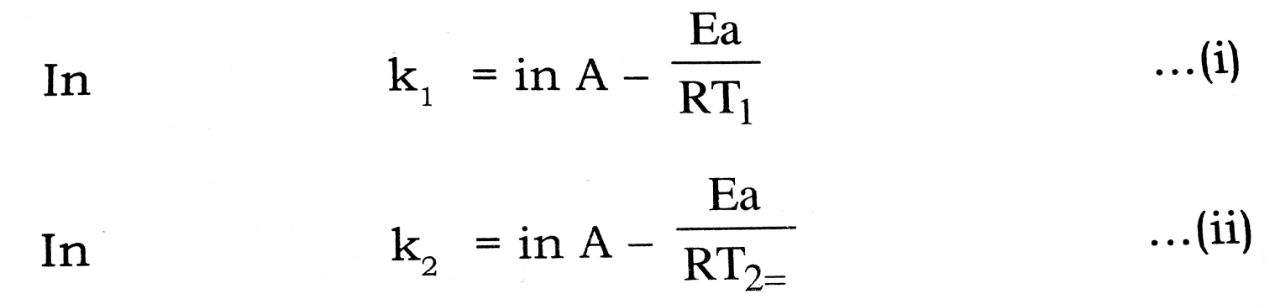Substacting eqn. (i) from eqn. (ii), we get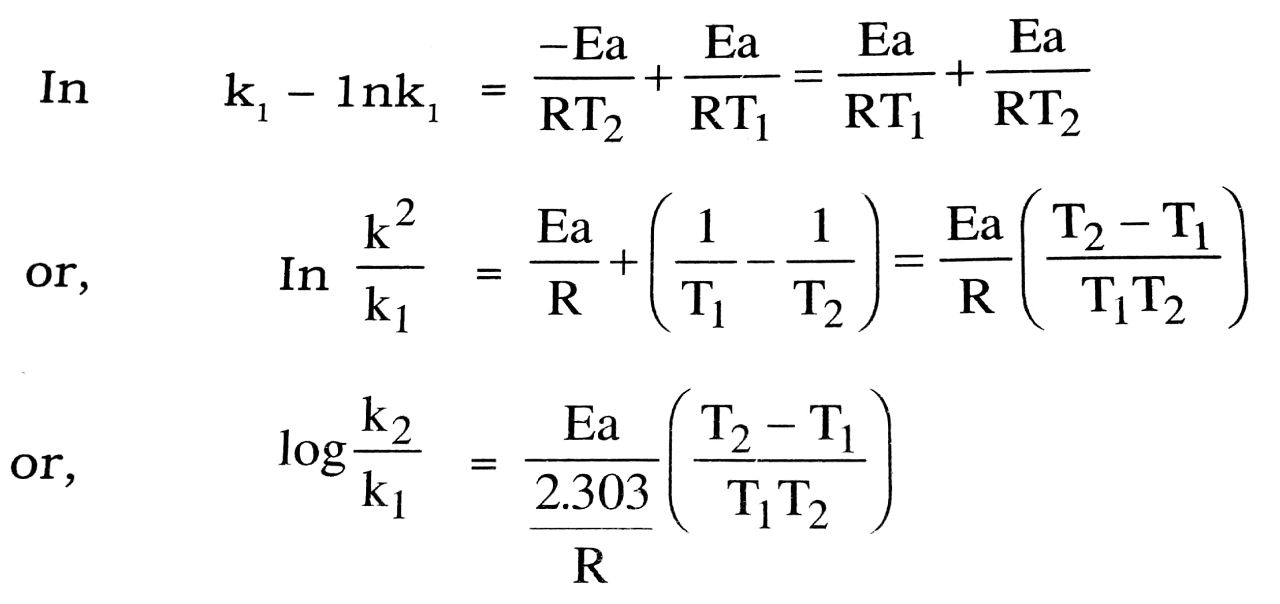Thus knowing the values of the constant k, and k2 at two different temperature T1 and T2, the value of Ea can be calculated.

Q. 9. (a) What do you mean by zero order reaction ? How the value of rate constant is determined ? What is the relation between rate constant and half-life period ?

(b) Give two examples.
(c) What are the factors on which the rate of reaction depends ? Discuss each factor in brief.

Ans⇒ (a) When the rate of the reaction is independent of the concentration of the reactants, the reaction is known as zero order reaction. In zero order reaction, the concentration of reactant (R) remains unaltered the course of reaction.

It means rate =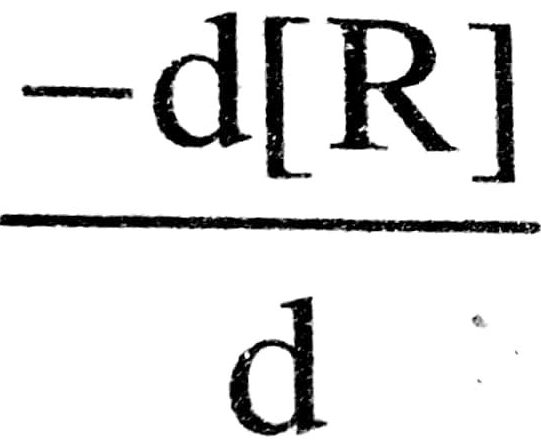= k[R]0 = k

or, d(R) = -kdt

On integration of equation we get

[R] = – kt + constant               ……(i)
Since [R] = [R]0

where t = 0 (i.e. on product is formed at the beginning be reaction), the constant must be zero.
Thus,                     [R]° = -K × 0 = constant

.                              Constant = [R]°

Put the value of constant in equn. (i) we get

[R] = kt + [R]°                         …. (ii)

When a plot is drawn between concentration of reactant (R) and time t, a straight line is obtained, slope of which gives the value of – k and intercept on y-axis is equal to the value of ‘[R]°.

Alternatively, the value of k can be obtained from egn. (i) by putting the value of concentration [R] at any time t and initial concentration. [R]°

Hence ,          k     =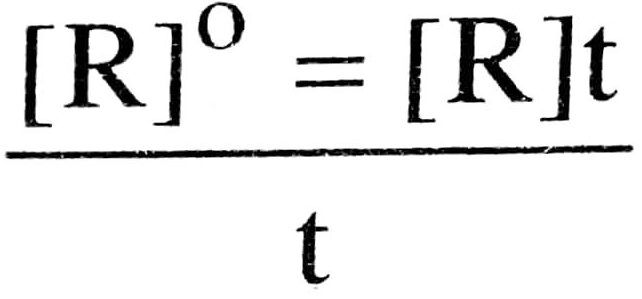Half life period is the time period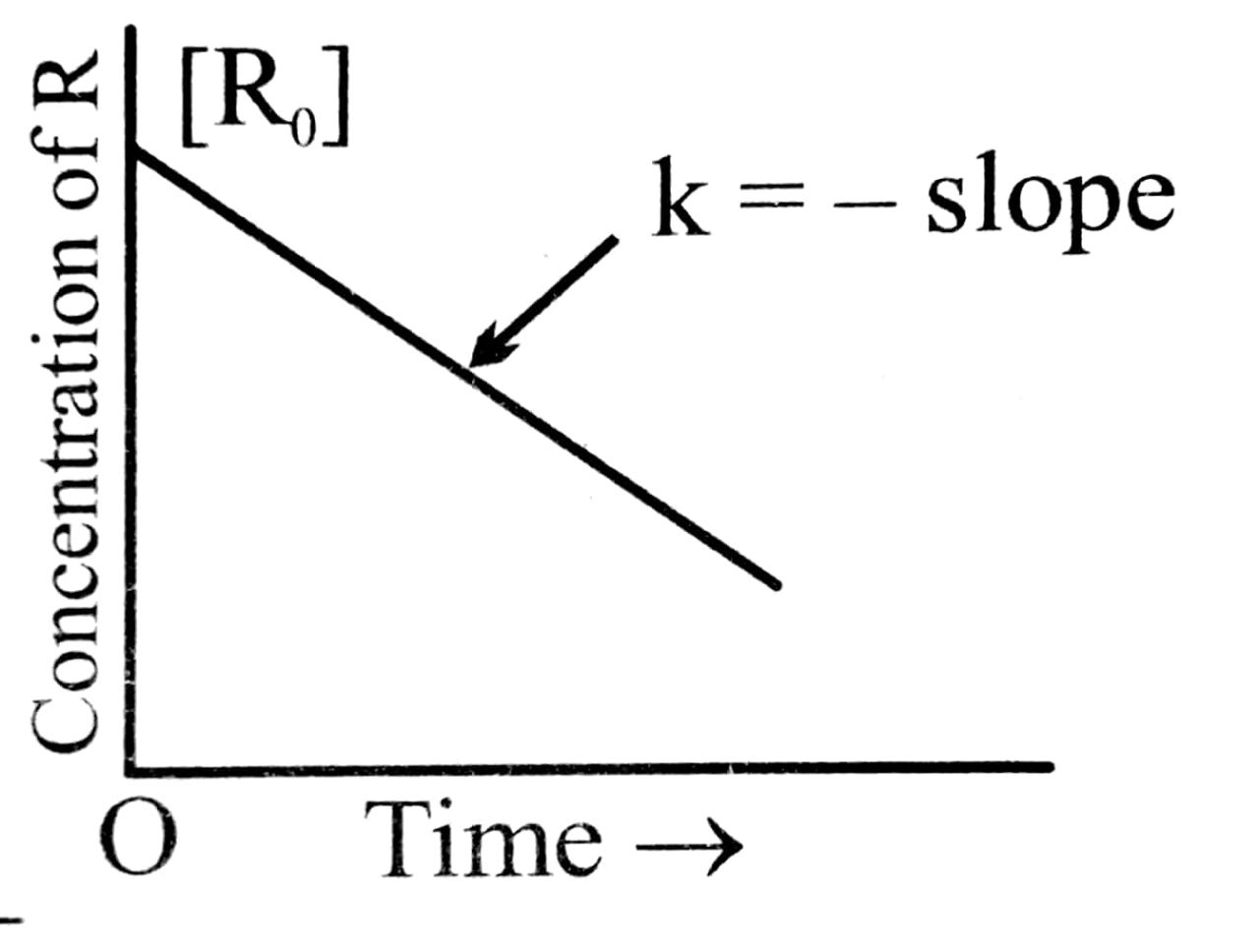to reduce the initial concentration
of the reactant to half of its initial value.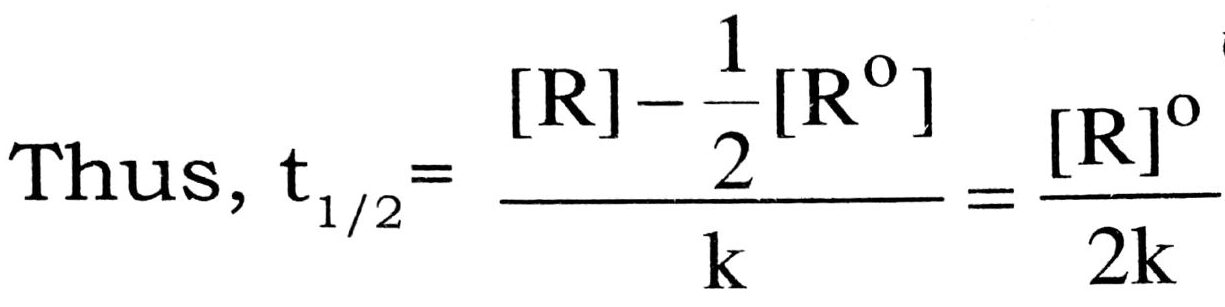Thus, the half life period is directly proportional to the initial concentration of the reactant, [R]° and inversely proportional to the rate constant k.

(b) Example : (i) Decomposition of NH3 on the surface of platinum.
(ii) Decomposition of HI on Gold surface.

(c) The rate of reaction depends upon the following factors :
(i) Concentration of the reactants : Greater the concentration faster is the reaction.

(ii) Presence of catalyst: It increases the speed of the reaction by lowering the activation energy.

(iii) Temperature : the rate of reaction increases with increase of temp, in most of cases, the rate of reaction become almost double for every 10°C rise in temp.

This is because of the increase in the number of effective collision with rise in temp.

(iv) Surface area : With increase in surface area rate of reaction increases.

(v) Nature of reactants: With change in reactants rate of reaction changes.

Q. 10. Define order and molecularity of reaction. Derive a general expression for specific rate constant of first order reaction.

Ans⇒ It is sum of the power of indicus of reacted terms no. of molecules which tke part in a chemical ribs known as molecularity of reaction.

Let us take a general exept soln. A → B
to, -0, a g moles/litre O.
‘t’-(a-x) g moles litre × g moles/litre.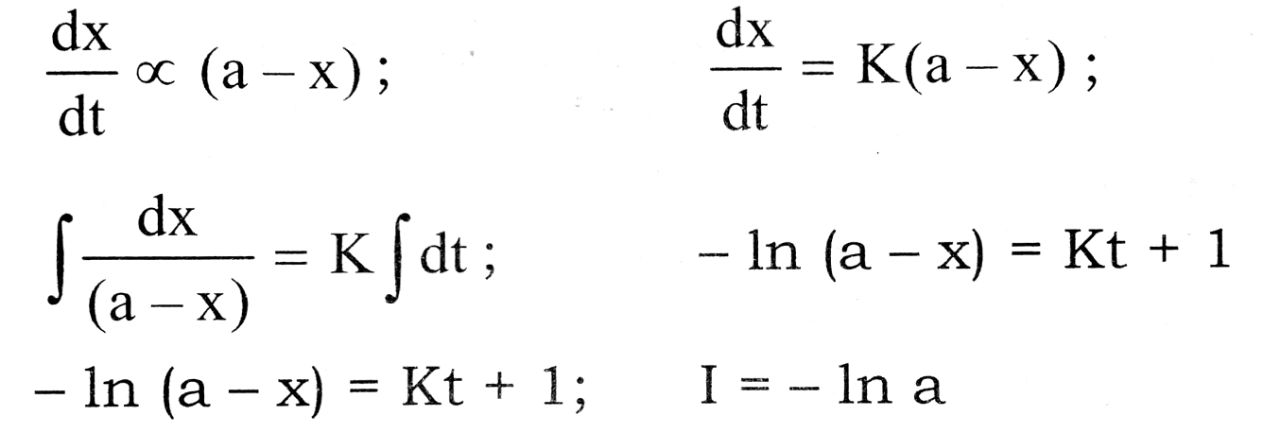ln (a – x) = kt – In a;        kt = ln a – lk (a – x)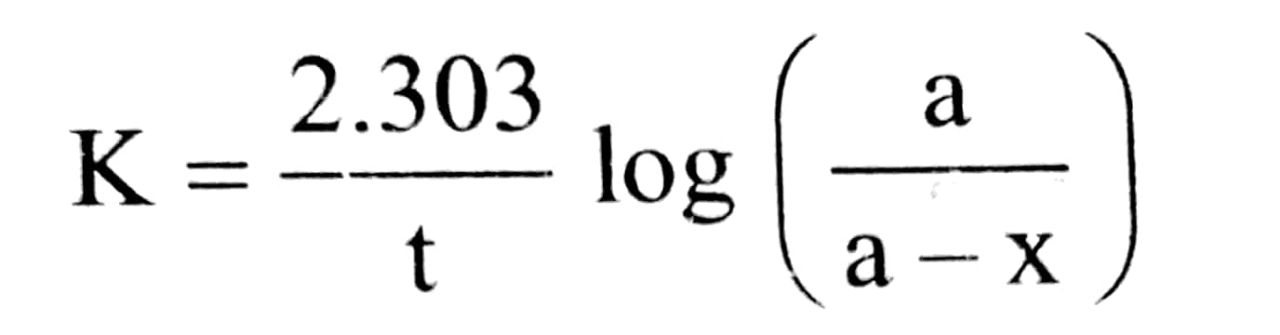Q. 11. (a) What is is the difference between Order and Molecularity of a reaction ?
(b) Rate of a reaction and Rate constant of a reaction ?
(c) What are the factors which influence the rate of reaction. Discuss them in detail.

Ans⇒ (a) Difference      between     Order    &   Molecularity :

 Molecularity Order 1. It is the number of reacting species undergoing simultaneous collision in the reaction. 1. It is sum of the power of the concentration terms in the rate law expression. 2. It is a theoretical concept. 2. It is determined experi- mentally. 3. It can have intergral values only. 3. It can have fractional values also. 4. It cannot be zero. 4. It can be zero. 5. It does not tell us anything about the mechanism of the reaction. 5. It tells us about the slowest step in the mechanism and hence gives some clue about mechanism of the reaction.

(b) Difference between Rate of a reaction and Rate constant :

 Rate of Reaction Rate constant of reaction 1. It is the speed at which the reactants are conveted into products at any moment of time. 1. It is a constant of proportonality in the rate law expression. 2. It depends upon the concentration of reactant species at that moment of time. 2. It refers to the rate of the reaction at the specific point when concentration of every reacting species is unity. 3. It decreases with the progress of reaction generally. 3. It is constant and does not depend on the process of the reaction. 4. It has same units for all reactions ML-1S-1 4. It has different units for different reactions.

(c) 1. Nature of the reacting species : Consider the following two reactions :

2NO (g) + O2 (g) → 2NO2 (g)                 …fast
2CO (g) + O2(g) → 2CO2 (g)                  …slow

These reactions appear to be similar but the first is fast while the second is slow. This is because different amounts of energies are required for breaking of different bonds and different amounts of energies are released in the formation of different bonds.

2. Concentration of the reactants : Greater are the concentrations of the reactants, faster is the reaction. Conversely, if the concentrations of the reactants decrease, the rate of the reaction also decreases. A match stick which burns usually in air (O2 : 21% by volume) burns with a flash in a jar containing O2 gas (volume of O2 : 100%)

3. Temperature : The rate of a reaction increases with the increase in temperature. In most of the reactions the rate of the reaction gets doubled for 10°C rise in temperature irrespective of the fact whether the reaction is exothermic or endothermic. In some cases reactions do not take place at room temperature but proceed only on heating

4. Surface area of the reactants : For a reaction involving a solid reactant or catalyst, the smaller is the particle size, i.e., greater is the surface area, the faster is the reaction. Wood shavings burn rapidly in air than a log of wood, because the surface area of wood shaving is much higher.

5. Pressure of a catalyst : A catalyst generally increases the speed of the reaction without itself being consumed in the reaction. In case of reversible reactions a catalyst helps attain chemical equilibrium quickly without disturbing the state of equilibrium. It speeds up the reaction both in the forward as well as backward direction so the equilibrium is attained earlier.

6. Presence of light : Some reactions do not take place in the dark, but take place in the presence of light.

e.g., H2 + Cl2 → 2HCl

Such reactions are called Photo-Chemical Reactions.

Q. 12. Calculate the half life of a first order reaction from their rate constants give below :

(a) 200 s-1 (b) 2 min-1 (c) 4 yers s-1

Ans⇒ For the first order reaction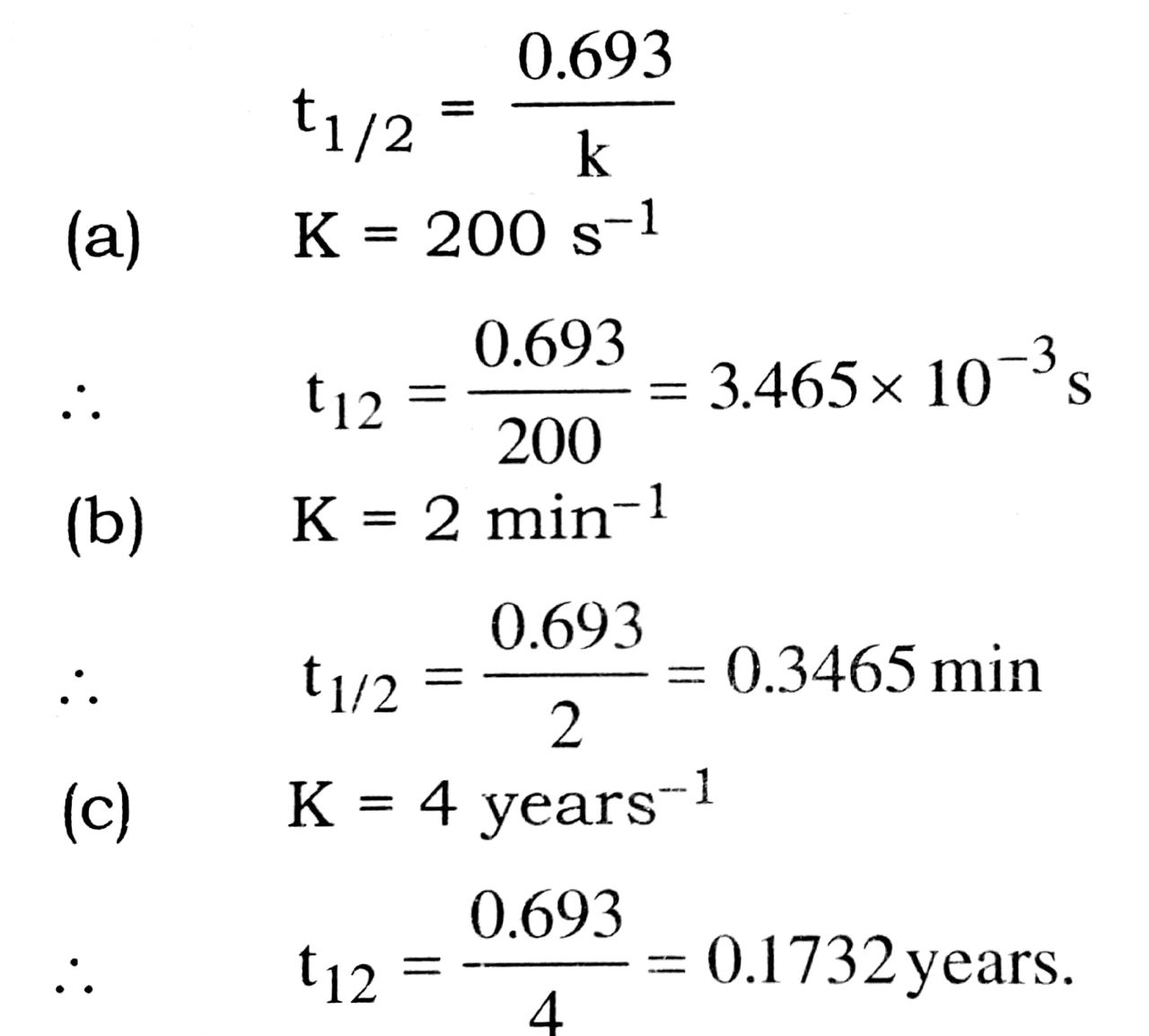Q. 13. The rate constant for a first order reaction is 60 s-1. How much time will it take to reduce the initial concentration of the reactant to its 1/16th value ?

Ans⇒ Rate constant of reaction,

k = 60 s-1; t15/16 = ?

Rate constant  of first order reaction is given bywhere 15/16th the reaction is over then

a – x = 1-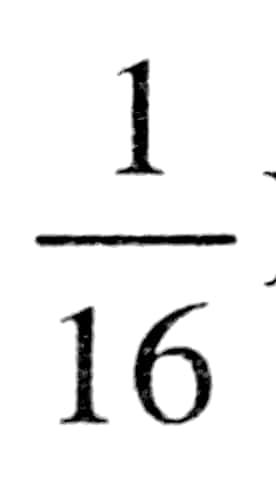Mifa = 1M.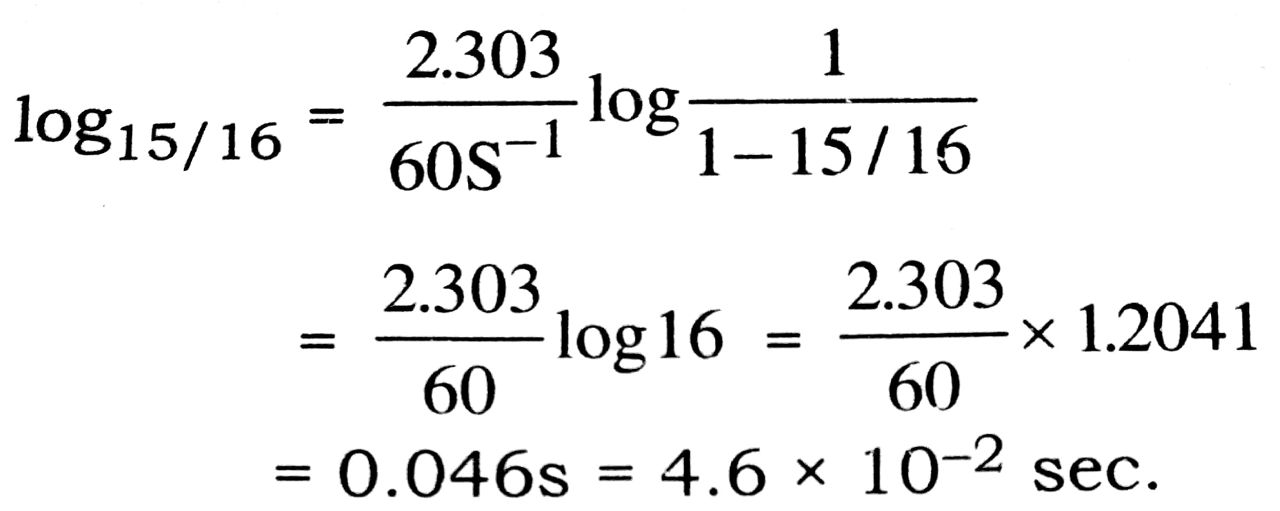Q. 14. A first order reaction takes 40 min for 30% decomposition. Calculate t1/2.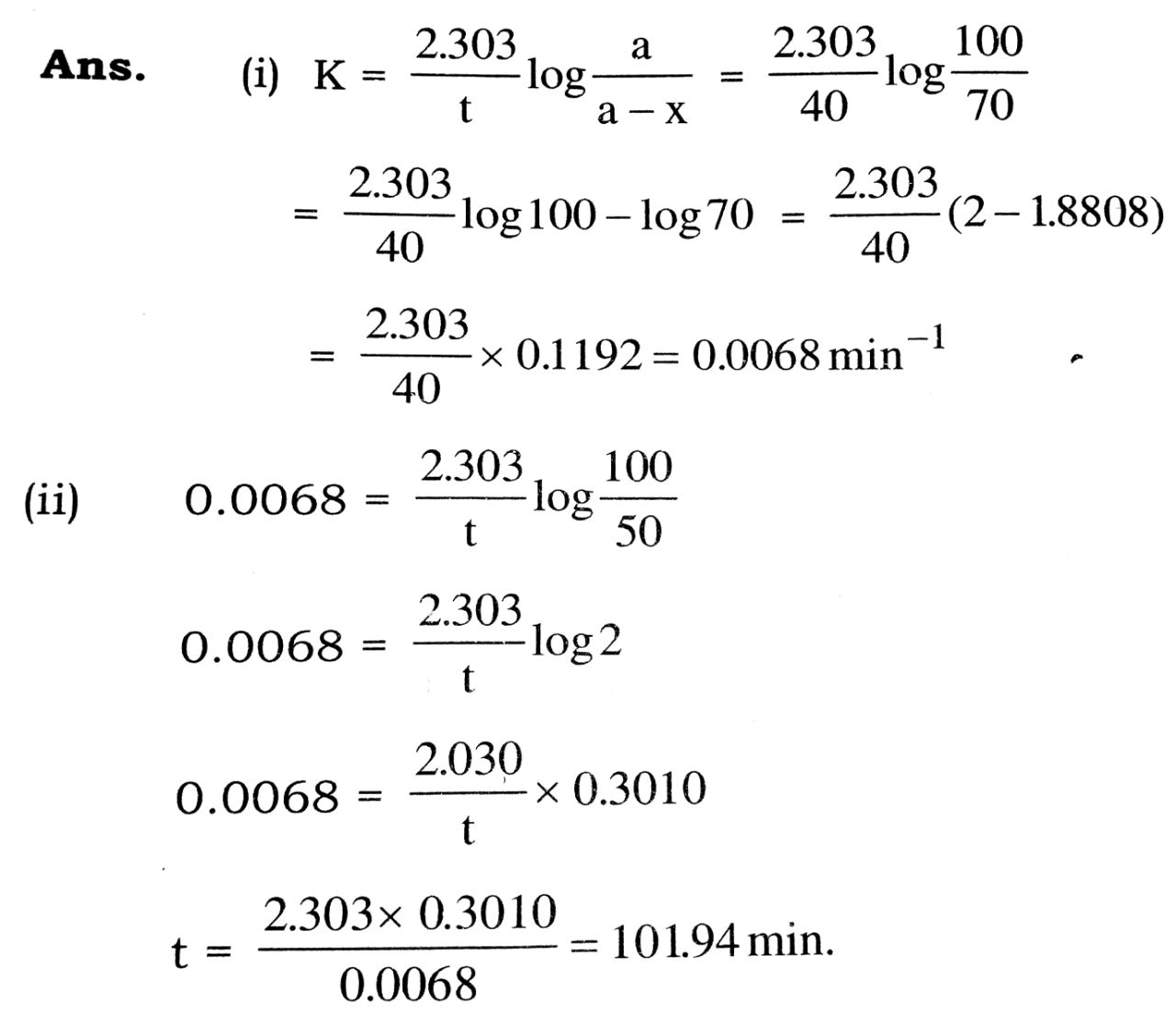## Class 12th Chemistry Long Type Question

 S.N CHEMISTRY LONG TYPE QUESTION 2022 1 SOLID STATE 2 SOLUTION 3 ELECTROCHEMISTRY 4 CHEMICAL KINETICS 5 SURFACE CHEMISTRY 6 GENERAL PRINCIPLES AND PROCESSES OF ISOLATION OF ELEMENTS 7 THE P-BLOCK ELEMENTS 8 THE D- AND f-BLOCK ELEMENTS 9 CO-ORDINATION COMPOUNDS 10 HALOALKANES AND HALOARENES 11 ALCOHOLS, PHENOLS AND ETHERS 12 ALDEHYDES, KETONES AND CARBOXYLIC ACIDS 13 AMINES 14 BIOMOLECULES 15 POLYMERS 16 CHEMISTRY IN EVERYDAY LIFE 17 DISTINGUISH BETWEEN PAIR 18 CONVERSIONS